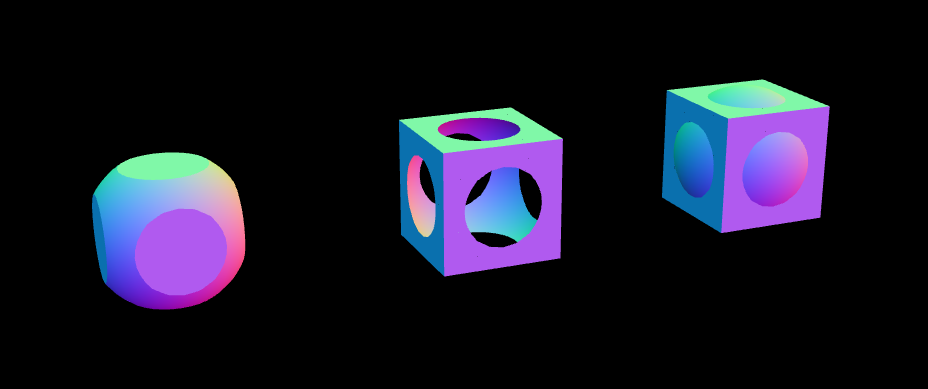# npm

## three-csg-ts3.1.13 • Public • Published

# three-csg-ts

CSG (Constructive Solid Geometry) library for three.js with Typescript support.

This is a typescript rewrite of THREE-CSGMesh.

Example## Concept

CSG is the name of a technique for generating a new geometry as a function of two input geometries.

CSG is sometimes referred to as "Boolean" operators in 3d modelling packages.

Internally it uses a structure called a BSP (binary space partitioning) tree to carry out these operations.

The supported operations are .subtract, .union, and .intersect.

By using different combinations of these 3 operations, and changing the order of the input models, you can construct any combination of the input models.

## Installation

• Install with npm npm i -save three-csg-ts
• Install with yarn yarn add three-csg-ts

## Example usage

import * as THREE from 'three';
import { CSG } from 'three-csg-ts';

// Make 2 meshes..
const box = new THREE.Mesh(
new THREE.BoxGeometry(2, 2, 2),
new THREE.MeshNormalMaterial()
);
const sphere = new THREE.Mesh(new THREE.SphereGeometry(1.2, 8, 8));

// Make sure the .matrix of each mesh is current
box.updateMatrix();
sphere.updateMatrix();

// Perform CSG operations
// The result is a THREE.Mesh that you can add to your scene...
const subRes = CSG.subtract(box, sphere);
const uniRes = CSG.union(box, sphere);
const intRes = CSG.intersect(box, sphere);

## Keywords

### Install

npm i three-csg-ts

### Repository

github.com/samalexander/three-csg-ts

3,160

3.1.13